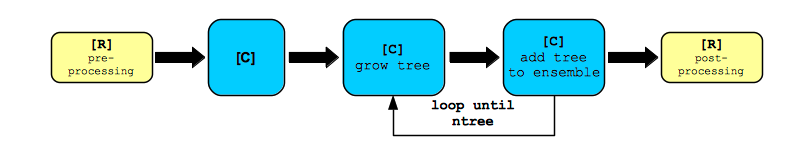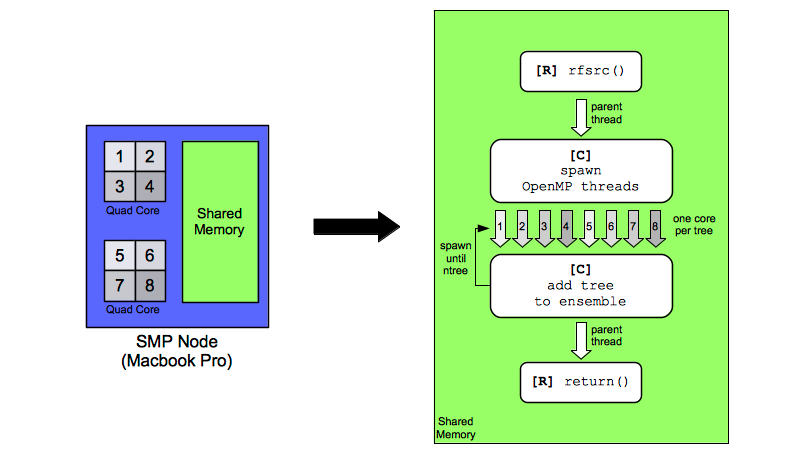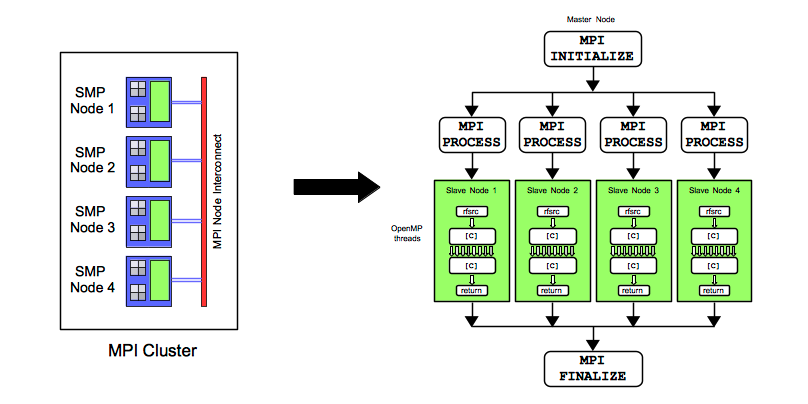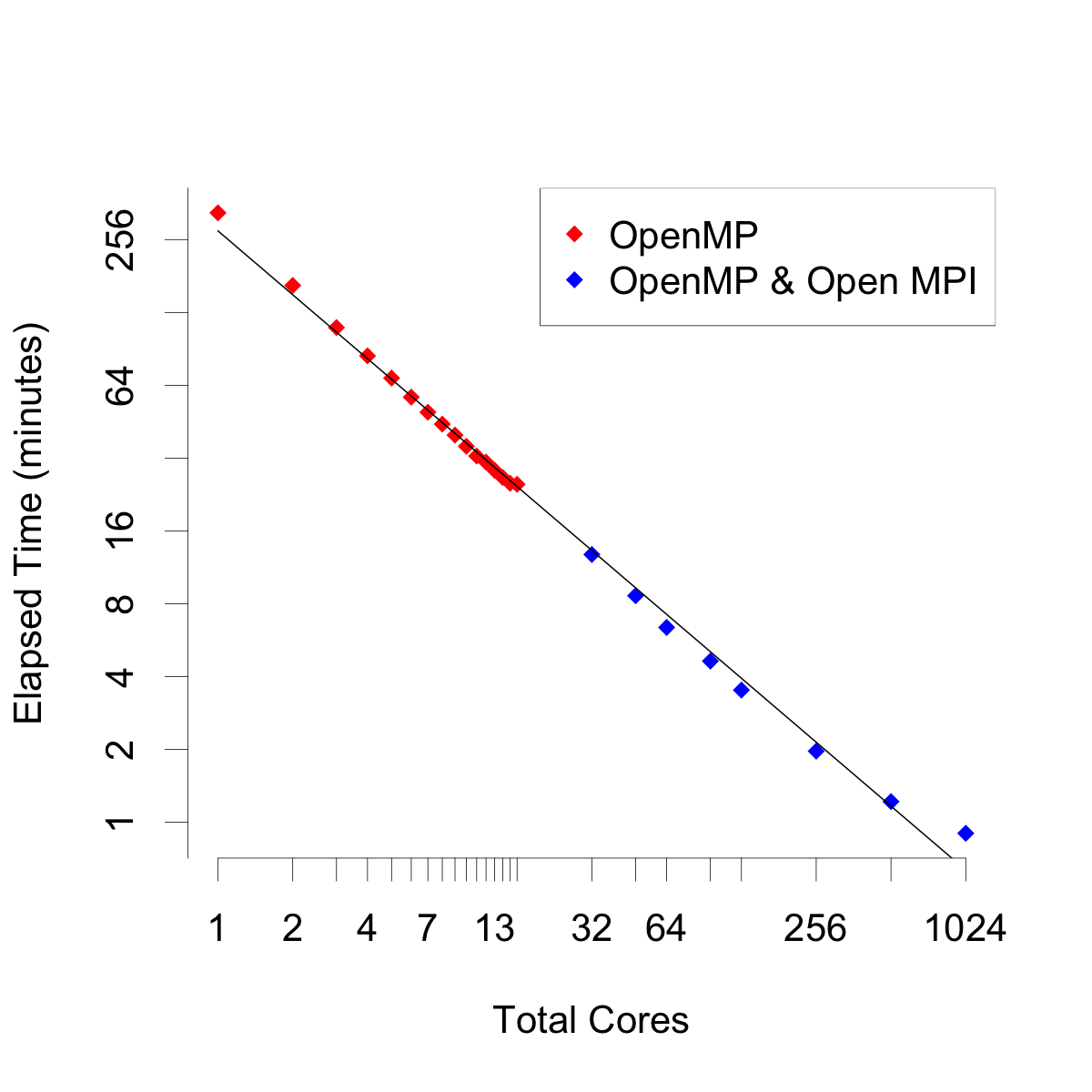This package has the capabilities of non-trivial parallel processing with massive scalability. Growing a forest has a recursive component as was shown in the Recursive Algorithm . But it is also an iterative process that is repeated ntree times. This fact is depicted in the figure Forest Growth as an Iterative Process. The entry point is a user-defined R script that initiates the grow call rfsrc(). This function pre-processes the data set, and calls a grow function in the package’s C library, which grows the forest. The C function grows a single tree, adds the resulting tree-specific ensemble(s) to the forest ensemble(s), and continues this process for ntree iterations. The C function then returns the forest ensemble(s) to the R function, which post-processes the results. It is advantageous to parallelize this iterative process. Such situations are often called “pleasingly parallel.” The default pre-compiled binaries, available on CRAN, should execute in parallel. If not, the package has been written to be easily compiled to accommodate parallel execution. As a prerequisite, the host architecture, operating system, and installed compilers must support it. OpenMP and Open MPI compilers must be available on the host system. If this is the case, it is possible to compile the source code to enable parallel processing.

###### Forest Growth as an Iterative ProcessA graphic showing one mode of parallel execution (OpenMP) on a typical desktop/laptop computer is shown in the figure OpenMP: Shared Memory Parallel Processing. In this example, the hardware on the left of the figure is a Macbook Pro (2015 model) with two quad-core CPUs, and 16GB of RAM. This hardware allows eight threads to execute simultaneously. The memory is shared among all cores, and this configuration is typical of symmetric multiprocessing (SMP) where two or more identical processors connect to a shared memory bank. Most reasonably configured multi-core notebooks and desktops are ideal hosts for randomForestSRC and OpenMP parallel execution. The right side of [Shared Memory Parallel Processing] shows the software implementation of the OpenMP model. The R code reads the data set, pre-processes the data set, calls the C code and requests a forest of ntree trees. When OpenMP parallel execution is in force, the C code grows trees simultaneously, rather than iteratively. Each core is tasked with growing a tree. Once a core completes growing a tree, the forest ensemble values are incremented with the contribution of that tree, and the core is re-tasked with growing another tree. This continues until the entire forest is grown. Control is then returned to the R code which does post-processing of the results. On the hardware shown in the figure, it is possible to grow eight trees at a time. Under ideal conditions, the elapsed time to grow a forest should decrease linearly and be close to a factor of eight.

###### OpenMP: Shared Memory Parallel ProcessingIn order to give the user some flexibility, core usage can be controlled via R options. First, the user needs to determine the number of cores on the machine. This can be done within an R session by loading the parallel package and issuing the command detectCores(). It is possible to set the numbers of cores accessed during OpenMP parallel execution at the start of every R session by issuing the command options(rf.cores = x), where x is the number of cores. If x is a negative number, the package will access the maximum number of cores on the machine. The options command can also be placed in the users .Rprofile file for convenience. Alternatively, one can initialize the environment variable RF_CORES in the user’s command shell environment.

Gains in performance on a desktop (or a single SMP node) are limited by the number of cores available on the hardware. The OpenMP parallel model shown in OpenMP: Shared Memory Parallel Processing that relies on an underlying iterative process was chosen for its suitability in accommodating shared memory systems and its ease of implementation. Model creation is computationally intense and the OpenMP parallel processing model allows us to take full advantage of the hardware available.

Message Passing Interface (MPI), an alternative model to OpenMP is suitable for distributed memory systems, in which the number of cores available can be much greater than that of a desktop computer. It is possible to run randomForestSRC on appropriately designed and configured clusters, allowing for massive scalability. An ideal cluster for the package is made up of tightly connected SMP nodes. Each SMP node has its own memory, and does not share this memory with other nodes. Nodes, however, do communicate with each other using MPI.

###### Hybrid: MPI/OpenMP Parallel ProcessingIn the figure Hybrid: MPI/OpenMP Parallel Processing, a generic cluster with four SMP nodes is depicted. Each node can be considered to be similar to a desktop computer. However, in a cluster setting, each node is tightly supervised and controlled by a top-level layer of cluster software that handles job scheduling, and resource management. On the left of the figure, four nodes are connected via a network that facilitates MPI communications across nodes. The right of the figure depicts the software implementation of hybrid parallel processing using the randomForestSRC package. A primary/replica framework is chosen whereby a primary node on the cluster is tasked with growing the forest. The primary node divides the forest into sub-forests. If the size of the forest is ntree, then each replica in this example is tasked with growing a sub-forest of size ntree/4. Hybrid parallel processing is dependent on the Rmpi package. This package must be available. If it is not, it must compiled and installed from the source code available on CRAN. The Rmpi is a mature package and provides flexible low level functionality. Other explicitly parallel general-use packages on CRAN such as snow, snowfall, and foreach provide higher level user friendly functions that, while potentially useful, were considered not as flexible and customizable in a cluster environment.

In the hybrid example that follows, Rmpi is loaded on the primary node, and MPI processes are then spawned on each replica. Disregarding the fact that the replicas can communicate with the primary using MPI, each replica acts like an SMP node in figure OpenMP: Shared Memory Parallel Processing. A replica initiates concurrent OpenMP threads across it cores, and grows its sub-forest using shared memory OpenMP parallel processing. It is possible to grow many more trees concurrently on a cluster, because the forest is spread among many more cores than are available on a single node, or desktop computer. In figure Hybrid: MPI/OpenMP Parallel Processing, the forest uses 32 cores concurrently. In an ideal environment, the elapsed time to grow the original forest will be reduced by a factor of 32. After all replicas have completed growing their sub-forests, they return their data to the primary process, via MPI messages, and the replicas terminate. On a single SMP node (equivalent to a desktop computer), a thread on a core starts, grows a tree, terminates, and repeats this process until all trees have been grown. A core is either waiting for a task, working on a task, or has completed a task. A task, in this case, is the growing of a tree. On a cluster, a replica node starts, grows a sub-forest, and terminates. It repeats this process until all sub-forests requested by the primary node have been grown. A replica is either waiting for a sub-forest, working on a sub-forest, or has completed a sub-forest.

As proof of concept, and for benchmarking purposes we ran the package on two clusters available to us. The first cluster was the Learner Research Institute High Performance Cluster (LRI-HPC) at the Cleveland Clinic. This system runs Simple Linux Utility for Resource Management (SLURM) supervisor software. It is a Linux-based cluster consisting of twenty nodes with ten cores per node. Each node has 64GB of shared memory. The second cluster was the Pegasus 2 installation at the High Performance Computing Group at the Center for Computational Science (HPC CCS) at the University of Miami. This system has 10,000 cores spread across over 600 nodes. The system also runs a flavour of Linux but uses Platform LSF as the job scheduler. The different job schedulers for the two clusters required minor customization of the calling shell scripts. For our benchmarks, we analyzed a simulated survival data set with n=3000, p=30, and ntree=1024, with the default value of importance="permute". We ran the package in serial mode and scaled up to 1024 cores across 64 nodes. The results are shown in this figure. In serial mode, growing 1024 trees iteratively (using one core) took about 330 minutes. The red points all reflect OpenMP parallel processing, on one node. These results would be similar to that of a desktop computer. Pegasus 2 has 16 cores per node. When using all cores on one node were used, elapsed time decreased to about 25 minutes. This reflects a decrease in a factor of 13 from the serial time. The theoretical maximum decrease would be closer to a factor of 16. The results would be similar to that of running the analysis on desktop computer with 16 cores. Next, hybrid parallel processing using the primary/replica framework was implemented. Two replica nodes were tasked, then three, and so on up to 64 nodes. At 64 nodes, total core usage was 1024. At this number, the cluster was growing all 1024 trees simultaneously. The data points reflecting hybrid parallel processing are in blue. Linear decreases in elapsed time were observed. This indicates that the package is capable of massive scalability. The primary and replica code harnesses are given in the section Supplementary Code.

###### Benchmark of Hybrid Parallel Processing### Supplementary Code

##### Generalized code for simulating the survival data set used in Benchmark of Hybrid Parallel Processing:
###########################################################################
# Survival Simulation (Cox)
###########################################################################

design.cov <- function(p, cov.x, equalCorr=F) {
if (!equalCorr){
x <- matrix(0,p,p)
for(i in 1:p){
x[i,(i:p)] <- cov.x^(0:(p - i))
x[i,(1:(i - 1))] <- x[(1:(i - 1)),i]
}
}
else{
x <- matrix(cov.x,p,p)
diag(x) <- 1
}
return(x)
}

mvngeneration <- function(n, p, varcov) {
## function used to generate mvn dist with given mean vector and variance
## covariance matrix (here mean vector is all zeros)
## n is the sample size
## p the dimension
choleski <- chol(varcov)
tcholeski <- t(choleski)
z <- matrix(rnorm(p * n, 0, 1), p, n)
tranx <- tcholeski %*% z
x <- t(tranx)
x
}

simmOneCox <- function (n, p, cov.x, s, signal) {
x <- mvngeneration(n, p, design.cov(p, cov.x))
beta.true <- rep(0, p)
x.center <- round((p-s)/2)
beta.true[x.center+(1:s)] <- signal
linPred <- x %*% beta.true
Time <- round(sapply(linPred, function(lp){rexp(n=1,exp(-lp))}), 2)
C <- round(rexp(n, exp(-mean(linPred))), 2)
dat <- data.frame(time=pmax(1e-3, pmin(Time,C)), event=as.numeric(Time<=C), x)
return(dat)
}

###########################################################################
# Regression & Classification Simulation
###########################################################################

simmOne <- function (n, p) {
x <- matrix(data = rnorm(n*p), nrow=n, ncol=p)
coeff <- c(1:p)
resp <- x %*% coeff
dat <- data.frame(outcome = resp, x)
return(dat)
}

get.data <- function() {

## Define the family.
family = c("surv", "regr", "class")

## IF CLASSIFICATION:
## Number of levels in classification response
c          <- 4

## IF SURVIVAL:
## Survival Simulation Constants
s <- 5
cov.x <- 0
signal <- c(0, 1)

## DIMENSIONS:
p          <- 300
n          <- 6000

if (family == "surv") {

sim.data.org  <- simmOneCox(n, p, cov.x, s, signal)

## f <- as.formula(Surv(time, event) ~ .)
} else {

sim.data.org  <- simmOne(n, p)

if (family == "class") {
outcome.cut <- cut(sim.data.org$outcome, breaks = c) sim.data.org$outcome <- outcome.cut
}

## f <- as.formula(outcome ~ .)
}

return (sim.data.org)

}
##### The primary harness used in Benchmark of Hybrid Parallel Processing:
##########################################################################
##  Primary Harness
##########################################################################

## Load the R package that implements MPI parallel processing.
require("Rmpi")
}

## Load our package on the master.
library(randomForestSRC)

## Set the slave and core usage.
RF_SLAVES =    4  ## Number of slaves.
MC_CORES  =    1  ## Turn off mclapply() in package:parallel.
RF_CORES  =   -1  ## Use all cores (and threads) on each slave.

## Create the simulated data set.
data.sphere <- get.data()

## Spread the forest evenly among the slaves.
ntree <- round(1000 / RF_SLAVES)

## Calculate importance.
importance <- "permute"

## Spawn the number of slaves we have specified.
mpi.spawn.Rslaves(nslaves = RF_SLAVES, needlog = TRUE)

## Cleanup resources taken up by Rmpi.
.Last <- function(){
if (mpi.comm.size(1) > 0){
print("Please use mpi.close.Rslaves() to close slaves.")
mpi.close.Rslaves(dellog = FALSE)
}
print("Please use mpi.quit() to quit R")
.Call("mpi_finalize")
}
}

## Meaningless message content.
junk <- 0

## Note the number of slaves actually spawned.  This should be the
## number requested, but we do not do any checks.
n_slaves <- mpi.comm.size() - 1

## Set the number of task equal to the number of slaves.  In general,
## the number of tasks can be greater ## than the number of slaves.
## In such a scenario, tasks are run in a simple round-robin fashion
## on the available slaves.

## Keep track of the number of closed slaves.
closed_slaves <- 0

## Create the task list. Note that we label them with their forest
## identifier.
}

## Crete a list to hold the resulting forests.

## Send the slave function to the slaves.
mpi.bcast.Robj2slave(rfslave)

## Send core usage information to the slaves.
mpi.bcast.Robj2slave(MC_CORES)
mpi.bcast.Robj2slave(RF_CORES)

## Send the data, and relevant variables to the slaves.
mpi.bcast.Robj2slave(data.sphere)
mpi.bcast.Robj2slave(ntree)
mpi.bcast.Robj2slave(importance)

## Load our package on the slaves.
mpi.bcast.cmd(library(randomForestSRC))

## Call the function in all the slaves. The function will idle on a
## slave until the master sends it a task.
mpi.bcast.cmd(rfslave())

## Continue processing tasks until all slaves have closed down.
while (closed_slaves < n_slaves) {

## Receive a message from a slave.
message <- mpi.recv.Robj(mpi.any.source(), mpi.any.tag())
message_info <- mpi.get.sourcetag()
slave_id <- message_info
tag      <- message_info

if (tag == 1) {
## tell it that all tasks are done if there are no more.
## Send a task, and then remove it from the task list.
}
else {
## Tell the slave that there are no more tasks.
mpi.send.Robj(junk, slave_id, 2)
}
}
else if (tag == 2) {
## The message from the slave contains results.  Extract the forest
## identifier, the forest object, and add it to the list object.
forest.id <- message$forest.id mresult <- list(forest.id = message$forest.id, outcome = message$outcome) result[[forest.id]] <- mresult } else if (tag == 3) { ## A slave has closed down. Make note of it. closed_slaves <- closed_slaves + 1 } } ## Combine the out-of-bag predicted results. combine.predicted.oob = 0 for (i in 1:n_tasks) { combine.predicted.oob <- result[[i]]$outcome$predicted.oob + combine.predicted.oob } combine.predicted.oob = combine.predicted.oob / n_tasks combine.class.oob <- apply(combine.predicted.oob,1, function(x) which.max(x)) combine.importance = 0 for (i in 1:n_tasks) { combine.importance <- result[[i]]$outcome$importance + combine.importance } combine.importance = combine.importance / n_tasks ## Detach our package on the slaves. mpi.bcast.cmd(detach("package:randomForestSRC", unload=TRUE)) ## Close down the slaves. mpi.close.Rslaves(dellog = FALSE) ## Detach our package on the master. detach("package:randomForestSRC", unload=TRUE) ## Terminate MPI execution and exit. mpi.quit() ##### The replica harness used in Benchmark of Hybrid Parallel Processing: ########################################################################## ## Replica Harness ########################################################################## rfslave <- function() { ## Note the use of the tag for sent messages: ## 1=ready_for_task, 2=done_task, 3=exiting ## Note the use of the tag for received messages: ## 1=task, 2=done_tasks junk <- 0 done <- 0 ## Set the core usage in the slave. options(mc.cores = MC_CORES) options(rf.cores = RF_CORES) ## Continue, as long as the task is not "done". Note the use of ## the tag to indicate the type of message. while (done != 1) { ## Tell the master we are ready to receive a new task. mpi.send.Robj(junk, 0, 1) ## Receive a new task from the master. task <- mpi.recv.Robj(mpi.any.source(),mpi.any.tag()) task_info <- mpi.get.sourcetag() tag <- task_info ## Determine whether we received a task from the master. if (tag == 1) { ## Perform the task, and create results. ## Extract the forest ID from the task. forest.id <- task$forest.id

## Grow a forest on the slave.
partial <- rfsrc(outcome ~ .,
data = data.sphere[, c(1,2,3,4)],
ntree=ntree,
importance = importance)

## Construct and send a message containing the resulting
## forest back to master.
result <- list(forest.id=forest.id, outcome = partial)
mpi.send.Robj(result, 0, 2)

}
else if (tag == 2) {
## The master indicates that all tasks are done. Exit the loop.
done <- 1
}
else {
## Unknown message.  Ignore it.
}
}

## Tell master that this slave is exiting.  Send an exiting message.
mpi.send.Robj(junk, 0, 3)
}

Cite this vignette as
H. Ishwaran, M. Lu, and U. B. Kogalur. 2021. “randomForestSRC: hybrid parallel processing vignette.” http://randomforestsrc.org/articles/hybrid.html.

@misc{HemantHybrid,
author = "Hemant Ishwaran and Min Lu and Udaya B. Kogalur",
title = {{randomForestSRC}: hybrid parallel processing vignette},
year = {2021},
url = {http://randomforestsrc.org/articles/hybrid.html},
howpublished = "\url{http://randomforestsrc.org/articles/hybrid.html}",
note = "[accessed date]"
}
1. Ishwaran H, Lu M, Kogalur UB. randomForestSRC: randomForestSRC algorithm vignette. 2021. http://randomforestsrc.org/articles/algorithm.html.